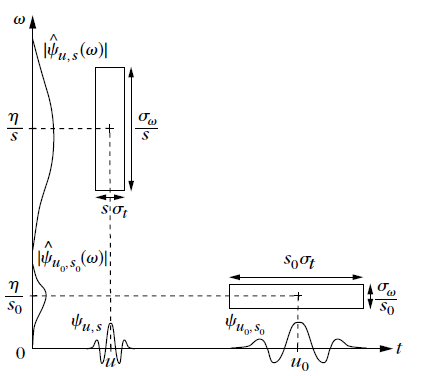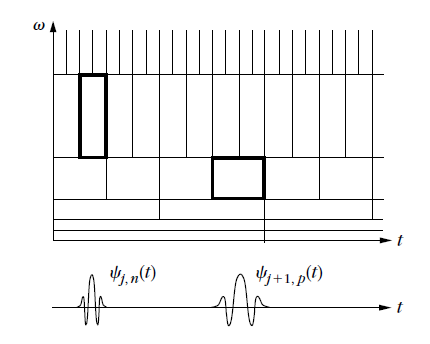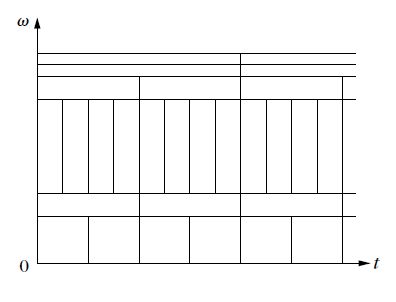# 0.4 Time-frequency dictionaries  (Page 3/3)

 Page 3 / 3

Large-amplitude wavelet coefficients can detect and measure short high-frequency variations because they have a narrow timelocalization at high frequencies. At low frequencies their time resolution is lower, but they have a better frequency resolution.This modification of time and frequency resolution is adapted to represent sounds with sharp attacks, or radar signals having afrequency that may vary quickly at high frequencies.

## Multiscale zooming

A wavelet dictionary is also adapted to analyze the scaling evolution of transientswith zooming procedures across scales. Suppose now that ψ is real. Since it has a zero average, a wavelet coefficient $Wf\left(u,s\right)$ measures the variation of $\phantom{\rule{0.4pt}{0ex}}f$ in a neighborhood of u that has a size proportional to s . Sharp signal transitionscreate large-amplitude wavelet coefficients.Heisenberg time-frequency boxes of two wavelets, ψ u , s and ψ u 0 , s 0 . When thescale s decreases, the time support is reduced but the frequency spread increases and covers an intervalthat is shifted toward high frequencies.

Signal singularities have specific scaling invariance characterized by Lipschitz exponents. Chapter 6 relates the pointwiseregularity of $\phantom{\rule{0.166667em}{0ex}}f$ to the asymptotic decay of the wavelet transform amplitude $|Wf\left(u,s\right)|$ when s goes to zero. Singularities are detected by followingthe local maxima of the wavelet transform acrossscales.

In images, wavelet local maxima indicate the position of edges, which aresharp variations of image intensity. It defines scale–space approximation support of $\phantom{\rule{0.166667em}{0ex}}f$ from which precise image approximations are reconstructed.At different scales, the geometry of this local maxima support provides contoursof image structures of varying sizes. This multiscale edge detection is particularly effective forpattern recognition in computer vision (Canny:86).

The zooming capability of the wavelet transform not only locates isolated singular events, but canalso characterize more complex multifractal signalshaving nonisolated singularities. Mandelbrot (Mandelbrot:82) was the first to recognizethe existence of multifractals in most corners of nature. Scaling one part of a multifractalproduces a signal that is statistically similar to the whole. This self-similarityappears in the continuous wavelet transform, which modifies the analyzing scale.From global measurements of the wavelet transform decay, Chapter 6 measuresthe singularity distribution of multifractals. This is particularly important in analyzing theirproperties and testing multifractal models in physics or in financial time series.

## Time-frequency orthonormal bases

Orthonormal bases of time-frequency atoms remove all redundancy and define stablerepresentations. A wavelet orthonormal basis is an example of the time-frequency basis obtained by scaling a wavelet ψ with dyadic scales $s={2}^{\phantom{\rule{0.166667em}{0ex}}j}$ and translating it by ${2}^{\phantom{\rule{0.166667em}{0ex}}j}n$ , which is written ${\psi }_{j,n}$ . In the time-frequency plane, the Heisenberg resolution box of ${\psi }_{j,n}$ is a dilation by ${2}^{\phantom{\rule{0.166667em}{0ex}}j}$ and translation by ${2}^{\phantom{\rule{0.166667em}{0ex}}j}n$ of the Heisenberg box of ψ . A wavelet orthonormal is thus a subdictionary of thecontinuous wavelet transform dictionary, which yields a perfecttiling of the time-frequency plane illustrated in [link] .The time-frequency boxes of a wavelet basis define a tiling of the time-frequency plane.

One can construct many other orthonormal bases of time-frequency atoms, corresponding to different tilings of the time-frequency plane.Wavelet packet and local cosine bases are two important examples constructed in Chapter 8, withtime-frequency atoms that split the frequency and the time axis, respectively, in intervals of varying sizes.

## Wavelet packet bases

Wavelet bases divide the frequency axis into intervals of 1 octave bandwidth. Coifman, Meyer, and Wickerhauser (CoifmanMW:92) havegeneralized this construction with bases that split the frequency axis in intervals of bandwidth that may be adjusted.Each frequency interval is covered by the Heisenberg time-frequency boxesof wavelet packet functions translated in time, in order to cover the whole plane, as shown by [link] .

As for wavelets, wavelet-packet coefficients are obtained with a filter bank of conjugate mirror filters that split the frequency axisin several frequency intervals. Different frequency segmentations correspond to different waveletpacket bases. For images, a filter bank divides the image frequency support in squares of dyadic sizes thatcan be adjusted.A wavelet packet basis divides the frequency axis in separate intervals of varying sizes. A tiling is obtainedby translating in time the wavelet packets covering each frequency interval.

## Local cosine bases

Local cosine orthonormal bases are constructed by dividing the timeaxis instead of the frequency axis. The time axis is segmented in successive intervals $\left[{a}_{p},{a}_{p+1}\right]$ . The local cosine bases of Malvar (Malvar:88) are obtainedby designing smooth windows ${g}_{p}\left(t\right)$ that cover each interval $\left[{a}_{p},{a}_{p+1}\right]$ , and by multiplying them by cosine functions $cos\left(\xi t+\phi \right)$ of different frequencies. This is yet another idea that has beenindependently studied in physics, signal processing, and mathematics.Malvar's original construction was for discrete signals. At the same time, the physicist Wilson (Wilson:87)was designing a local cosine basis, with smooth windows of infinite support, to analyze theproperties of quantum coherent states. Malvar bases were also rediscovered and generalizedby the harmonic analysts Coifman and Meyer (CoifmanM:91). These different views of the same bases brought tolight mathematical and algorithmic properties that opened new applications.

A multiplication by $cos\left(\xi t+\phi \right)$ translates the Fourier transform ${\stackrel{^}{g}}_{p}\left(\omega \right)$ of ${g}_{p}\left(t\right)$ by $±\xi$ . Over positive frequencies,the time-frequency box of the modulated window ${g}_{p}\left(t\right)cos\left(\xi t+\phi \right)$ is therefore equal to the time-frequency box of g p translated by ξ along frequencies. [link] shows the time-frequency tiling corresponding to such alocal cosine basis. For images, a two-dimensional cosine basis is constructed by dividing theimage support in squares of varying sizes.

Got questions? Join the online conversation and get instant answers!By OpenStaxBy Katie MontroseBy Tamsin KnoxBy Mary MateraBy Michael PittBy OpenStaxBy Eric CrawfordBy Richley CrapoBy Rohini AjayBy Hannah Sheth